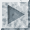[an error occurred while processing this directive]

## pseudoprimepseudoprime /n./ A backgammon prime (six consecutive occupied points) with one point missing. This term is an esoteric pun derived from a mathematical method that, rather than determining precisely whether a number is prime (has no divisors), uses a statistical technique to decide whether the number is `probably' prime. A number that passes this test was, before about 1985, called a `pseudoprime' (the terminology used by number theorists has since changed slightly; pre-1985 pseudoprimes are now `probable primes' and `pseudoprime' has a more restricted meaning in modular arithmetic). The hacker backgammon usage stemmed from the idea that a pseudoprime is almost as good as a prime: it does the job of a prime until proven otherwise, and that probably won't happen.